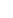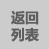### 选型直线：134-2489-9492您的位置：首页>新闻中心>常见问题## 冷却塔流量怎么算，看完就会！78.6×1.2 = 94.32 m3/h(每小时的水流量)

100×1.1=110 吨水/小时

316000×1.25(恒值)= 395000 Kcal/h,

1.25——冷凝器负荷系数

395000÷5 = 79000 KG/h = 79 m3/h

79×1.2(余量) = 94.8m3/h(冷却塔水流量)

(电制冷主机—通式：匹数×2700×1.2×1.25÷5000 或 冷吨×3024×1.2×1.25÷5000= 冷却塔水流量m3/h)

a.冷却水量=主机制冷量(KW)×1.2×1.25×861/5000(m3/h)

b.冷却水量=主机冷凝器热负荷(kcal/h)×1.2/5000(m3/h)

c.冷却水量=主机冷凝器热负荷(m3/h)×1.2(m3/h)

d.冷却水量=主机制冷量(冷吨)×0.8(m3/h)

e.冷却水量=主机蒸发器热负荷(kcal/h)×1.5×1.25/5000(m3/h)

f.冷却水量=主机蒸发器热负荷(m3/h)×1.2×1.25(m3/h)

g.冷却水量=主机蒸发器热负荷(冷吨)×1.2×1.25×3024/5000(m3/h)

Q=cm(T2-T1)t是时间,即降温需要多少时间

Q单位J;冷却塔C比热,如果是水就是4.2kJ/K*kg ;T2-T1就是降温差值2018-12-14

### 相关产品

####0769-82999860